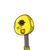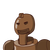# A and B can do a piece of work in 15 days. B and C can do the same work in 10 days and A and C can do the same work in 12 days. Ti

A and B can do a piece of work in 15 days. B and C can do the same work in 10 days and A and C can do the same work in 12 days. Time taken by A, B and C together to do the job is?

### 2 thoughts on “A and B can do a piece of work in 15 days. B and C can do the same work in 10 days and A and C can do the same work in 12 days. Ti”

1.Step-by-step explanation:

Here First we find efficiency of the work done by A, B and C :-

A + B = 15

B + C = 10

C + A = 12

LCM of 15, 10, 12 will give it 60 ( Which is Total Work Done )

So efficiency of A + B is = 60 / 15 = 4 —–( 1 )

B + C is = 60 / 10 = 6 —–( 2 )

C + A is = 60 / 12 = 5 —–( 3 )

So Adding (1) (2) and (3) eq-n. We get :-

2 ( A + B + C ) = 15

A + B + C = 15/2 Ans.

So time taken by A B and C to do this job in a day is 7. 5.

2.LET ‘A’ IS CAPABLE OF DOING THE ENTIRE WORK INDIVIDUALLY IN X DAYS.

SIMILARLY LET ‘B’ AND ‘C’ COMPLETE INDIVIDUALLY IN ‘Y’ AND ‘Z’ DAYS.

THEN ( 10/X) + (10/Y) = 1; i.e 10{(X+Y)/XY = 1 ———————- (1)

SIMILARLY 12(Y+Z)/YZ = 1 ———————————————— (2)

AND 15(Z+X)/XZ = 1 ———————————————————-(3)

REWRITING (1/X)+(1/Y) = (1/10) ————————(4)

(1/Y) + (1/Z) = (1/12) ——————————————-(5)

(1/X ) + (1/Z) = 1/15 ——————————————(6)

SOLVING THE SIMULTANEOUS EQUATIONS

‘X’ = 24, ‘Y’ = 120/7, ‘Z’ = 40

IF A,B AND C ARE WORKING TOGETHER THE WORK THAT COULD BE COMPLETED IN A SINGLE DAY

= (1/X) + (1/Y) + (1/Z) = (1/24) + (7/120) + (1/40) = (5+7+3)/120 = 15/120 = 1/8

THE NUMBER OF DAYS REQUIRED TO COMPLETE THE WORK = 1/*1/8) = 8